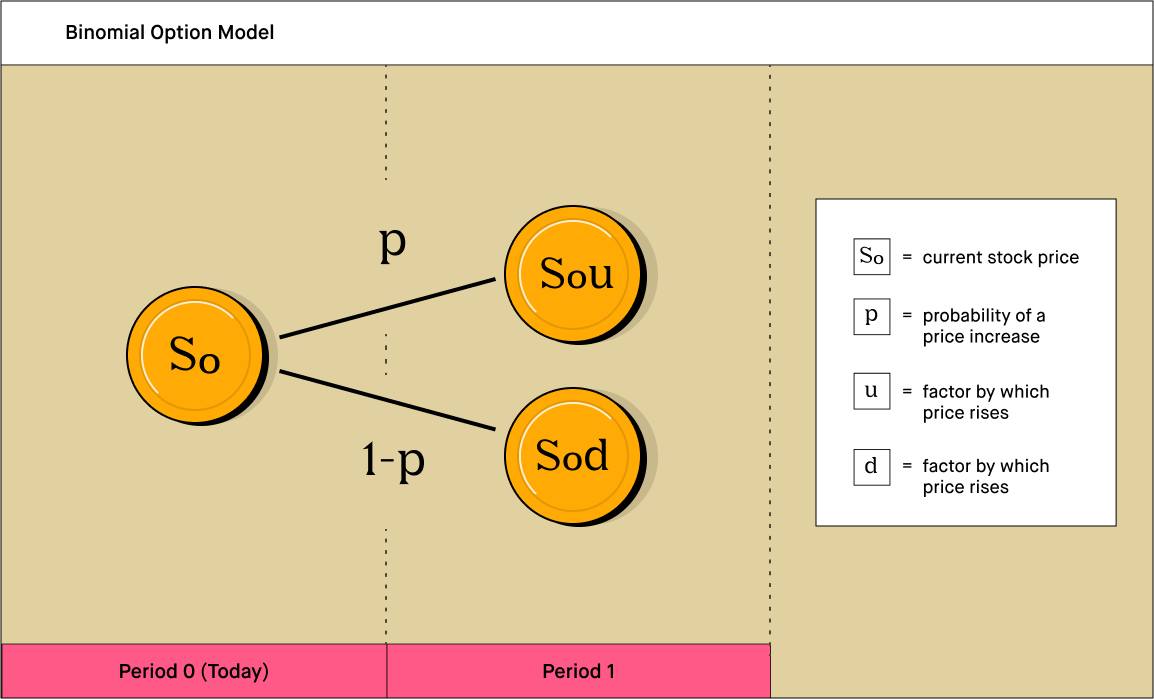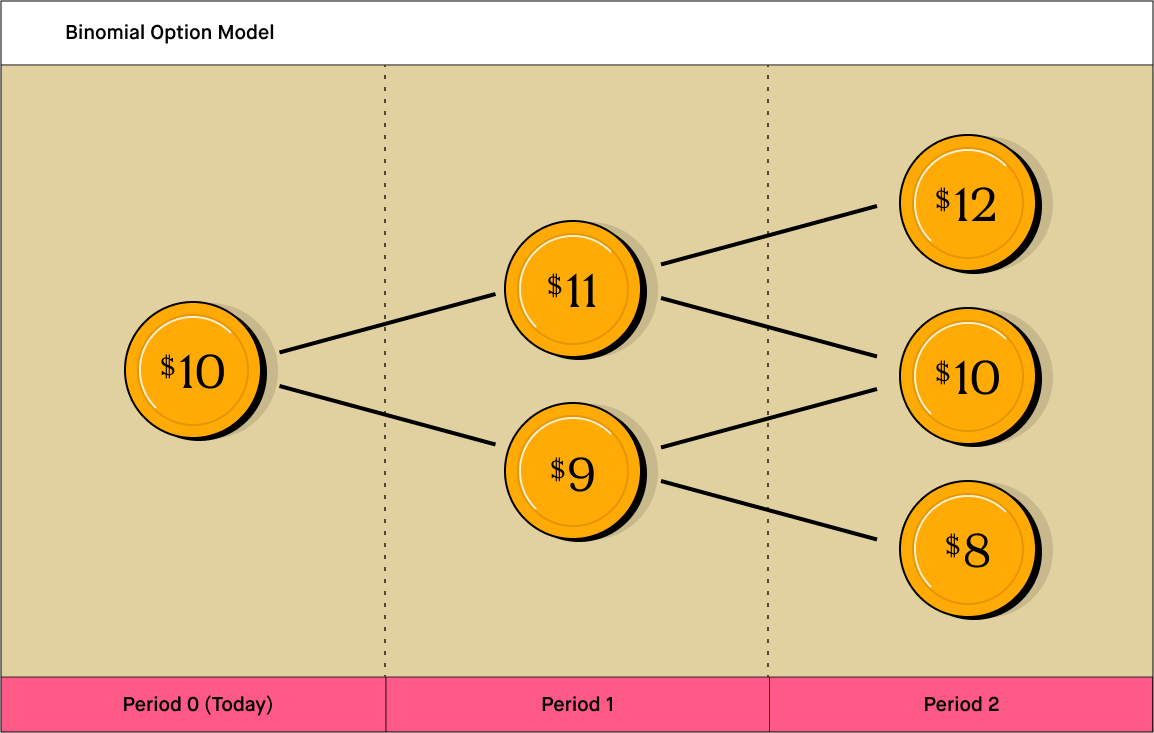# What is the Binomial Option Pricing Model?Robinhood Learn
Democratize finance for all. Our writers’ work has appeared in The Wall Street Journal, Forbes, the Chicago Tribune, Quartz, the San Francisco Chronicle, and more.
Definition:

The binomial option pricing model is a way for investors to evaluate options — the right to buy or sell specific securities at specified prices — over time.

## 🤔 Understanding the binomial option pricing model

The binomial options pricing model provides investors a tool to help evaluate stock options. It assumes that a price can move to one of two possible prices. The model uses multiple periods to value the option. The periods create a binomial tree — In the tree, there are two possible outcomes with each iteration. These outcomes are a move up or down the tree. To value the option, an investor needs to know the current price of a stock and the strike price of the option. The investor also needs to know the expiration date of the option.

Example

An investor is trying to determine the value of a stock option. The current stock price for fictitious Company Z is \$100, and the strike price is also \$100. For purposes of this example, the expiration date is in one year. The investor is confident that at the end of the year, the stock price will either be \$120 or \$80. They predict there is a 55% chance that the stock will be \$120, and a 45% chance that it will be \$90. The investor will use this information in the binomial options pricing model to find out what the current value of the option should be.

## Takeaway

The Binomial options pricing model is like a series of coin tosses...

When you toss a coin, you have two possible outcomes, heads or tails. Likewise, the binomial options model says there are two possible outcomes in every iteration. Of course, unlike in a coin toss, the probabilities in each iteration of the binomial options pricing model are not set at 50/50 — the probabilities can change in every time period based on new information.

Certain limitations apply

The free stock offer is available to new users only, subject to the terms and conditions at rbnhd.co/freestock. Free stock chosen randomly from the program’s inventory. Securities trading is offered through Robinhood Financial LLC.

Tell me more…

## What is the binomial option pricing model?

A binomial option pricing model is a pricing technique for options created in 1979. It follows an iterative method that helps evaluate prices over time. In the case of using optimization approaches based on minimizing an error function, the process starts with an initial guess and is optimized during iteration stages. then creates a series of closer estimates to determine a final value. In the case of the binomial model, an investor will know the current stock price. The investor then must guess what the stock price will be at the option expiration date. This model requires that there be two possible stock prices at the expiration date and a probability for each price.

At any point in time, the option price could go up or down. The model will split time between now and the expiration date into a series of periods, like weeks or months. At each step in the model, the stock price will go up or down. The model creates a binomial distribution of possible stock prices for the option. Think of it as possible paths that the stock price could go before the expiration date.

## Why is it the binomial option pricing model significant?

The binomial option pricing model is significant because it is easier to use than other models. You can compare the option price to the underlying stock prices of the option. It allows an investor to look at different periods for an option to the point of the expiration date. This benefit makes it a useful tool to value American style stock options. It is possible to exercise an American style option at any time until the options expiration date.

Also, the model simplifies things by assuming no arbitrage. Arbitrage is a short-term investment strategy. It occurs when an investor tries to profit by purchasing a security while also selling that security at a higher price. When there is no arbitrage, the value of your assets will always be the same. If you assume no-arbitrage in an option model, this allows you to value your option at each period. An investor can see the changes in prices in the underlying stock change from each period to the next. This transparency allows them to determine the best time to exercise the option.

One significant advantage is the multi-period view and its simplicity. The model provides an overview of the underlying stock’s price in different periods. It also provides transparency into the options underlying value over these periods. A trader could see the underlying stock price at each period and compare it to the change in the option price. An investor could also incorporate different probabilities into each step. The model is much more accurate for American style options. This is due to the ability to value the option over different periods. You can exercise American style options at any time before they expire. This creates a considerable advantage over other pricing models.

One major disadvantage of the model is that it takes longer to value the option. The calculations will take longer than other models if you're looking at many options. So, it is not very useful if you want to calculate a lot of options quickly. A key limitation of all pricing models is that the actual prices of options contracts are determined by market forces, not by formula no matter how sophisticated the formula may be.

## What is the difference between Black-Scholes and binomial?

The Black-Scholes model is a different method for valuing options. The model evaluates price variances over time to help determine the price of an option. The model calculates the value with fixed inputs. These include the current stock price, the expiration date, the strike price, the risk-free rate, and the volatility. The binomial model provides a way to calculate the option over multiple periods.

Investors use the Black-Scholes model for European style options, which can only be exercised on their expiration dates. They use the binomial model for American style options. The Black-Scholes implies that the option has one correct value at the time of valuation. The binomial model calculates how the value of the option will change as time goes on. The model doesn’t imply one current value — It provides multiple possible values over time.

The Black-Scholes model is more of a black box. You enter inputs and get a result. The binomial model gives you transparency to see the prices over time. This transparency provides more applications for the model. For instance, you could enter different probabilities into each period.

## What is the key assumption of the binomial option pricing model?

The key assumption for the binomial model is that there are only two possible results for the stock. The two possible outcomes are a higher or a lower price. The price will go up, or it will go down. The probabilities are also an assumption. You will need to assume the likelihood of the two stock prices at the end of the period. Keep in mind that it is not possible to predict which way the price will go with any certainty. All investments carry risks.

To determine the outcomes, you need to know the current price, strike price, and expiration date. You must also assume a risk-free interest rate that will stay constant. When you have these values, you can calculate the option value for each period. With this information, you can determine the value of an option for a single period. You could also determine the value of an option over multiple periods.

## How do you calculate with the binomial option pricing model?

The first step in the calculation is to create a binomial tree. This tree will have a specified amount of time that ends at the expiration date. Each point on the tree is a node.Next, you need to calculate the valuations of each period. Start at the beginning with the current stock price and the strike price. Determine two possible stock prices at the end of the period. As an example, the current price is \$10, and the two possible prices are \$12 and \$8. Let’s say the strike price for a call option was \$10 as well.

Then move on to the next period. In this example, the period could be a month. The following month would include those two prices. Each of these prices will have two possible outcomes. For example, the results from \$12 could be \$10 and \$8. The outcomes from \$8 could be \$10 and \$6.

At each node, you could then determine the option value. Let’s go back to the first month when the stock price was \$10, and the option strike price was \$10. If the price increased to \$12, the option is worth \$2. If the value decreased to \$8, it is worthless.## How do you use the binomial option pricing model?

The binomial option pricing model is useful for traders to help estimate the theoretical values of options. Price movements of the underlying stocks provide insight into the values of options. The model provides a calculation of what the price of an option could be worth today. You could then compare that with the cost of the underlying stock.

If a trader can estimate the value of an option, it can help them in making trading decisions. If an investor owns an option and wants to find the best time to exercise it, he or she can calculate the value. The model also has other applications outside of valuing options. For instance, it can provide an estimate for any project with multiple periods. The model calculates the probability of success for anything with two different outcomes.

The above examples are intended for illustrative purposes only and do not reflect the performance of any investment. Investing involves risk, which means - aka you could lose your money. Keep in mind options trading entails significant risk and is not appropriate for all investors. Certain complex options strategies carry additional risk. To learn more about the risks associated with options trading, please review the options disclosure document entitled Characteristics and Risks of Standardized Options, available here or through https://www.theocc.com. Investors should consider their investment objectives and risks carefully before trading options. Supporting documentation for any claims, if applicable, will be furnished upon request.

The free stock offer is available to new users only, subject to the terms and conditions at rbnhd.co/freestock. Free stock chosen randomly from the program’s inventory. Securities trading is offered through Robinhood Financial LLC.

1203851

## Related Articles

What is a Strike Price?
Updated December 08, 2020

## You May Also Like

The 3-minute newsletter with fresh takes on the financial news you need to start your day.
The 3-minute newsletter with fresh takes on the financial news you need to start your day.